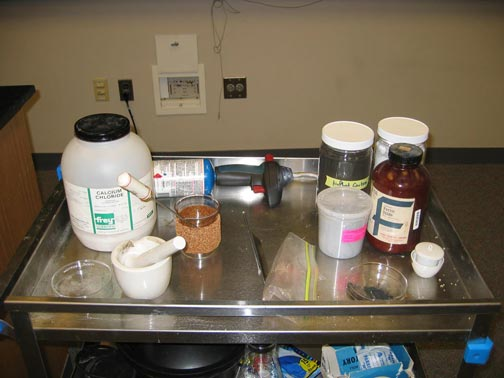### Exploring molar heats of reactionA constant quantity of heat is produced by two reactions using two very different quantities of reactants.

Ingredients: calcium chloride, ferric oxide, aluminum powder, black powder

Procedure: A complete recipe follows.

1. Prepare the thermite reaction using 1.0 gram of aluminum powder with 3.0 grams of ferric oxide powder.

2. Prime and ignite the thermite mixture, observing the heat evolved in the reaction.

3. Prepare the dissolution reaction of calcium chloride using 21.6 grams of salt.

4. Dissolve the salt and observe the heat evolved in the reaction.

5. Appreciate that the heat added to the surroundings in each case is the same.

Understanding: We have explored the highly exothermic thermite reaction

2 Al(s) + Fe2O3(s) → 2 Fe(s) + Al2O3(s)       ΔHothermite = -851.5 kJ

This is the most exothermic reaction that we will explore, one that produces molten iron at a temperature of 3000C!

Here is a way to appreciate just how exothermic the thermite reaction is. In a reaction of 1 gram of aluminum powder, roughly 16,000 joules (or 16 kilojoules) of heat is produced. Suppose that we wanted to produce the same amount of heat from the dissolution of calcium chloride salt in water

CaCl2 → Ca2+(aq) + 2 Cl-(aq)       ΔHodissolution = -81.35 kJ

which is a fairly exothermic dissolution reaction. What quantity of calcium chloride salt will be required?

We want to equate the total heat produced in each reaction. The heat produced in each reaction will be the molar enthalpy of reaction times the number of moles of reactant

qthermite = (mAl/2 MAl) ΔHothermite = qdissolution = (mCaCl2/MCaCl2) ΔHodissolution

where mAl is the mass of aluminum used in the thermite reaction, MAl=26.98 g/mol is the molar mass of aluminum, mCaCl2 is the mass of calcium chloride to be dissolved, and MCaCl2=110.984 g/mol is the molar mass of calcium chloride.

If we fix the amount of aluminum in the reaction to be mAl=1.0 g, the mass of calcium chloride that will be dissolved to produce the same amount of heat as the thermite reaction will be mCaCl2=21.6g. To produce 16 kilojoules of heat from the dissolution of calcium chloride used in hot packs, 21.6 grams of calcium chloride powder must be dissolved in water!

### Constant heats of reaction

Question: Consider the dissolution of magnesium sulfate. What is the mass of magnesium sulfate that would be dissolved to create the same heat of reaction as the thermite reaction? What about the dissolution of table salt? What is the mass of sodium chloride that would be dissolved to create the same heat of reaction as the thermite reaction?